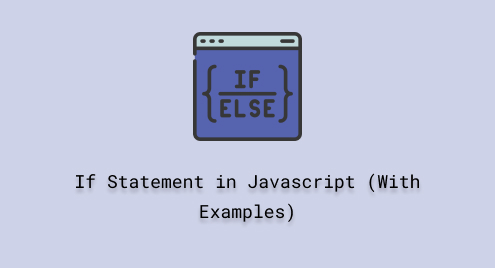# If Statement in Javascript (With Examples)

JavaScript “if” statement is an essential control flow component that permits you to run a code block based on a particular condition. It allows programs to take/handle decisions and choose different execution paths based on the evaluation of conditions that can be either true or false.

This blog will explain the “if” statement in JavaScript.

## What is “if” in JavaScript and How to Utilize it?

The “if” is a control flow or a conditional statement. It permits you to run a code block with respect to a certain event. It is a key component in the JavaScript programming language.

Syntax

Follow the provided syntax for utilizing the “if” statement:

```if (condition) {
// Code block to execute if the condition is true
}```

According to the provided syntax, here, the “condition” is a test expression that can be evaluated as “true” or “false”. If the specified condition is “true”, the code within the curly braces “{ }” will run otherwise the code block will be skipped and the program moves towards the next statement/block of code.

## Example 1

Here, we will see how the “if” statement block is executed. Create a variable “num” and assign a value “2”:

`let num = 2;`

In the “if” statement we will check whether the number is even or odd using the modulus “%” operator in the condition:

```if (num % 2 == 0){
console.log("Even Number");
}```

The output indicates that the if condition is true so the block is executed: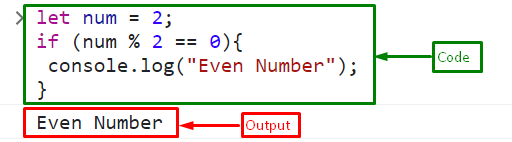## Example 2

Here, we will use the “else” clause with the “if” statement that will execute when the condition of the “if” statement is “false”:

```let num = 5;

if (num % 2 == 0){
console.log("Even Number");
}
else{
console.log("Odd Number");
}```

The output indicates that the if condition is false so the block of else statement is executed: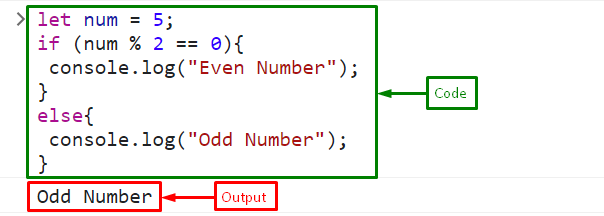## Example 3

In the given example, we will use multiple conditions using the “else if” condition. If the first condition of the “if” statement is “false” then the “else if” condition will be checked:

```if (num % 2 == 0){
console.log("Even Number");
}
else if (num % 2 != 0){
console.log("Odd Number");
}```

Output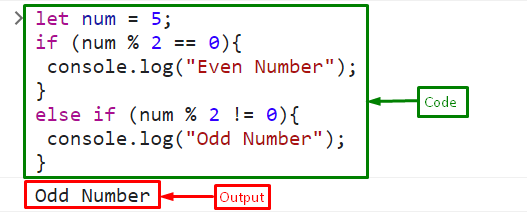## Example 4

In the following example, we will use the nested “if” conditions which allow to check multiple conditions and execute different blocks of code accordingly:

```let num = 25;

if (num > 10) {
if (num % 2 === 0) {
console.log("The number is even");
} else {
console.log("The number is odd");
}
}
else{
console.log("The number is less than 10");
}```

Output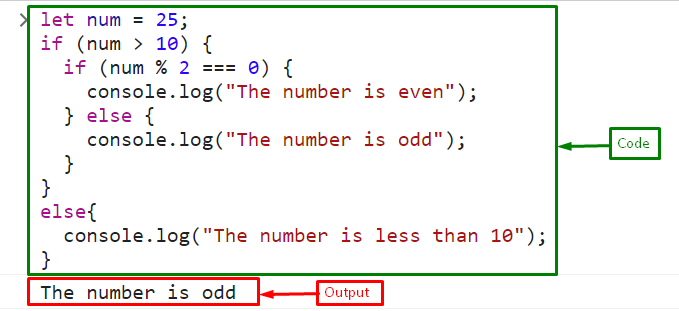That was all about the “if” statement in JavaScript.

## Conclusion

The “if” statement is a conditional statement that allows the execution of a code block depending on a certain condition. It is used to perform simple checks or create complex decision structures with nested if statements, the if statement provides the flexibility to handle various scenarios in JavaScript code. This post explained the JavaScript “if” statement.